Deletion - Linked list as we know for performing operations on the linked list we need to find the reference to the node of the list. so to delete a node from any position in the linked list we need to first find the reference of the node.

and if you don't know how to find the reference of a node in the linked list then first complete read this post.

## Deletion - Linked list in Data structures and algorithms

1. Deletion of the first node
2. Deletion of the only node
3. Deletion in between the node
4. Deletion at the end of the list

### Deletion of the first node from the linked list.

as you see we have a linked list that contains 5 items in it.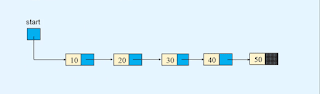to delete the first node of the list we need to point the start variable of the linked list to the second node of the list.so now the first node of the linked list is deleted.```def delete_first_node(self):
if self.start is None:

### Deletion of the only node from the linked list.

like if we have a list that has only one node.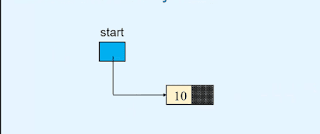then to delete the only node of the list we store the null or None value to the start variable.so now our list becomes empty.`self.start = None`

### Deletion in between the nodes of a linked list

to delete a node between the nodes of the linked list first we need to find the reference of the predecessor of the node that we want to delete.
like in the example given below we want to delete the second and fourth nodes. so we want to delete the node that contains the value 30.
so we need to find the reference to the node that comes before the node that contains the value 30.then we refer the p node to the node that comes second after the node p. as you see in the image given below.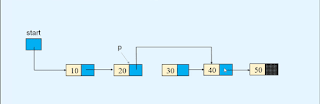now the node is deleted from the list.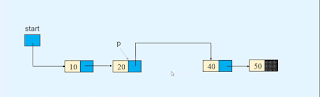### Deletion at the end of the linked list.

to delete the last node we need a reference to the second last node of the linked list. as we see in the image given below.after that, we set the linked part of p to null or None.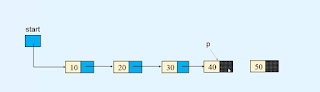so now the last node of the linked list is deleted.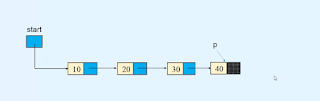```def delete_last_node(self):

if self.start is None:
return
self.start = None        return
p = self.start

#### Python program for traversing in the linked list.

```class Node:

def __init__(self, value):
self.info = value

def __init__(self):
self.start = None
def display_list(self):
if self.start is None:
print("List is empty")
return        else:
print("List is :   ")
p = self.start
while p is not None:
print(p.info, " ", end=' ')
print()

def count_nodes(self):
p = self.start
n = 0        while p is not None:
n += 1            p = p.link
print("Number of nodes in the list = ", n)

def search(self):
position = 1        p = self.start
while p is not None:
if p.info == x:
print(x, " is at position ", position)
return True            position += 1            p = p.link
else:
return False
def insert_in_beginning(self, data):
temp = Node(data)
self.start = temp

def insert_at_end(self, data):
temp = Node(data)
if self.start is None:
self.start = temp
return
p = self.start

def create_list(self):
n = int(input("Enter the number of nodes  :  "))
if n == 0:
return        for i in range(n):
data = int(input("Enter the element to be inserted : "))
self.insert_at_end(data)

def insert_after(self, data, x):
p = self.start
while p is not None:
if p.info == x:

if p is None:
print(x, "not present in the list")
else:
temp = Node(data)

def insert_before(self, data, x):
# If list is empty        if self.start is None:
print("List is empty")
return
if x == self.start.info:
temp = Node(data)
self.start = temp
return

p = self.start

print(x, " not present in the list")
else:
temp = Node(data)

def insert_at_position(self, data, k):
if k == 1:
temp = Node(data)
self.start = temp
return
p = self.start
i = 1        while i < k - 1 and p is not None:
i += 1
if p is None:
print("You can insert only upto position", i)
else:
temp = Node(data)

def delete_node(self, x):

if self.start is None:
print("List is empty")

if self.start.info == x:
return
p = self.start

print("Element ", x , "not in list")
else:

def delete_first_node(self):
if self.start is None:

def delete_last_node(self):

if self.start is None:
return
self.start = None            return
p = self.start
def reverse_list(self):

prev = None        p = self.start
while p is not None:
prev = p
p = next
self.start = prev

def bubble_sort_exdata(self):

end = None
p = self.start
if p.info > q.info:
p.info, q.info = q.info, p.info
end = p

end = None
r = p = self.start
if p.info > q.info :
if p != self.start:
else:
self.start = q
p,q = q,p
r = p
end = p

def has_cycle(self):
if self.find_cycle() is None:
return False        else:
return True
def find_cycle(self):
if self.start is None or self.start.link is None:
return None
slowR = self.start
fastR = self.start

while fastR is not None and fastR.link is not None:
if slowR == fastR:
return slowR
return None
def remove_cycle(self):
c = self.find_cycle()
if c is None:
return        print("Node at which the cycle was detected is ", c.info)

p = c
q = c
len_cycle = 0
while True:
if p == q:
break        print("Length of cycle is :", len_cycle)

len_rem_list = 0        p = self.start
while p != q:

print("Number of nodes not included in the cycle are : ", len_rem_list)
length_list = len_cycle + len_rem_list
print("Length of the list is : ", length_list)

p = self.start
for i in range(length_list-1):

def insert_cycle(self, x):
if self.start is None:
return        p = self.start
px = None        prev = None
while p is not None:
if p.info == x:
px = p
prev = p

if px is not None:
else:
print(x, " not present in list")

def merge2(self, list2):
merge_list.start = self._merge2(self.start, list2.start)
return merge_list

def _merge2(self, p1, p2):
if p1.info <= p2.info:
startM = p1
else:
startM = p2
pM = startM

while p1 is not None and p2 is not None:
if p1.info <= p2.info:
else:

if p1 is None:
else:

return startM

def merge_sort(self):
self.start = self._merge_sort_rec(self.start)

def _merge_sort_rec(self, list_start):

if list_start is None or list_start.link is None:
return list_start

start1 = list_start
start2 = self._divide_list(list_start)
start1 = self._merge_sort_rec(start1)
start2 = self._merge_sort_rec(start2)
startM = self._merge2(start1, start2)

return startM

def _divide_list(self, p):
while q is not None and q.link is not None:

##########################

list.create_list()

while True:
print("1. Display list")
print("2. Count the number of nodes")
print("3. Search for an element")
print("4. Insert in empty list/insert in beginning of the list")
print("5. Insert a node at the end of the list")
print("6. Insert a node after a specified node")
print("7. Insert a node before a specified node")
print("8. Insert a node at a given position")
print("9. Delete first node")
print("10. Delete last node")
print("11. Delete any node")
print("12. Reverse the list")
print("13. Bubble sort by exchanging data")
print("14. Bubble sort by exchanging links")
print("15. Merge sort")
print("16. Insert Cycle")
print("17. Detect Cycle")
print("18. Remove Cycle")
print("19. Quite")

if option == 1:
list.display_list()
elif option == 2:
list.count_nodes()
elif option == 3:
data = int(input("Enter the element to be searched : "))
list.search(data)
elif option == 4:
data = int(input("Enter the element to be inserted : "))
list.insert_in_beginning(data)
elif option == 5:
data = int(input("Enter the element to be inserted : "))
list.insert_at_end(data)
elif option == 6:
data = int(input("Enter the element to be inserted : "))
x = int(input("Enter the element after which to insert : "))
list.insert_after(data, x)
elif option == 7:
data = int(input("Enter the element to be inserted : "))
x = int(input("Enter the element before which to insert : "))
list.insert_before(data, x)
elif option == 8:
data = int(input("Enter the element to be inserted : "))
k = int(input("Enter the position at which to insert : "))
list.insert_at_position(data, k)
elif option == 9:
list.delete_first_node()
elif option == 10:
list.delete_last_node()
elif option == 11:
data = int(input("Enter the element to be deleted : "))
list.delete_node(data)
elif option == 12:
list.reverse_list()
elif option == 13:
list.bubble_sort_exdata()
elif option == 14:
elif option == 15:
list.merge_sort()
elif option == 16:
data = int(input("Enter the element at which the cycle has to be inserted : "))
list.insert_cycle(data)
elif option == 17:
if list.has_cycle():
print("List has a cycle")
else:
print("List does not have a cycle")
elif option == 18:
list.remove_cycle()
elif option == 19:
break    else:
print("Wrong option")
print()```